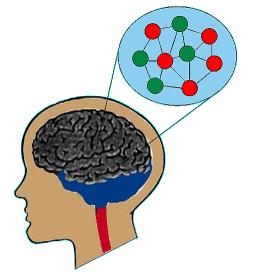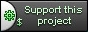Neural Entity[Home]
[Up]
[Presentation]
[Definitions]
[Object Structure]
[Evaluation Procedure]
[Learning Procedure]
[Create Pattern]

6. Create Pattern

## Purpose

The creation of a pattern is a research of a neural interconnection that will fit the data I/O constraints (we call here the constraints the expected output result required for specific inputs).

Most of the neural networks are first designed (choice of the neurons and the network type - these choice are the result of experience from the designer), then, the only processing left is to find an ad hoc dendrite's weights that will fit the constraints. We notice the interconnection between neurons to be a rigid one once the type of neural network chosen: there is not much creation left... the creativity reside mostly in the choice of the network type.

With our neural entity solution, we soon realize it is primordial to choose the type and the amount of connection carefully as a different connection type, for example the AND neural entity may become an OR or even an EOR with a small change. Just like a classical neural network, a part of the neural solution resides in the choice of interconnection between neuron; the fitting of the dendrites' weights are merely the fine tuning of a solution. The only difference resides in the amount of possible interconnection between two neurons and that if possible with a couple of input for one output become unfeasible for a bigger network (even a 4 inputs for 2 outputs would already become unfeasible). Also for each input set we may find several interconnection that will fit the constraints, so which one to choose?

Due to the amount of solutions and as a solution is to be adapted with time, we have to automated this creation process: the pattern creation is necessary to welcome situation where the amount of constraints increase with time and a solution that once fit the constraints may require a total different new approach to embrace new constraints. When we were adapting once the weights to fit a mathematical solution, we are here redesigning our solution (i.e. choosing a better neural interconnection) to better fit our growing constraints set.

## Logic

The logic used in the pattern creation is in fact quite simple as concept. We have a couple of rules to respect and from there work it out. The rules are:

1. Each input neuron is not necessary part of the solution: a neuron input could be superfluous for the solution and therefore must analyzed and eventually omitted in the search of a solution. This implies the following rule...
2. At least one input neuron must be part of the solution. That should be a certainty: the choice of the input neuron should be carefully chosen. Avoid too many but do not be too restrictive: this choice must be the result of a previous small analysis.
3. An input neuron may be an indirect part of the solution. In fact, one input neuron may alter the flow of another input neuron that is directly or indirectly connected to the output solution.
4. Each input neuron may have several connection, each of them possibly of different type. A neuron can be connected to one or several neuron, each neuron connection could be single or multiple.
5. There is no redundancy of connection type between two neurons. It is quite pointless to have two same type of connection between the same neuron.

The logic to find a pattern fitting the constraints is as followed:

1. Consider all possible combination of neurons with at least on output neuron in the solution. We will always start considering two neurons, one input and one output.
2. For each input neuron consider all combination of connection between this neuron an any other neurons taken into account in the previous step. At the situation where we have two inputs for one output, consider that each input chosen may be connected to two neuron: one output and one input.
3. For each connection between two neurons, evaluate each type of connection (Cf. Evaluation Procedure). Gradually evaluate the impact of one change at a time.
4. A specific type of connection requires a mode deeper analysis: when a synaptic knob is connected to another synaptic knob (Cf. Object Structure). As the neuron this connection will affect may have several other connections, each combination of them have to be evaluated gradually.

## Estimation of evaluation

We will mainly concentrate on the worse case (Murphy's case: the acceptable solution is always the last one tested whatever you choose to start with).

Let us have for example a couple of these values according to the amount of constraints. We will name  a n/m constraints a set of n inputs and m outputs.

 Constraints # combinations # Types combination 2/1 8 1,212 2/2 48 64,428 3/1 124 > 2,000,000 3/2 2196 ????????

We do not need to waste much time to understand the functions as is need an urgent improvement in order to be possibly usable.

In that perspective, I will overview the different option I will analyze for a potential improvement of the process.

## Weight of a Neural Pattern

In order to chose between several potential patterns, we will have to associate the pattern with a value. The 'best' pattern will be the one minimizing the weights. Simply considered, the more a neuron will be important in the solution, the more its weight will be. Ironically, we cold see a virtually weightless pattern with a lot of input neuron and a heavy weight pattern with few input neurons.

The weight of a neural pattern must take into account the weight of each neuron and the weight of the pattern.

### Weight of a Neuron

We will have to take the following into account:

1. The number of connections: the more connections, the heavier.
2. The type of connections.
3. The number of neuron it is connected (as a neuron may have up to several connections to a target neuron).
4. Point out some specialized neurons: like reducers (neuron connected only to soma), inhibitors (neuron connected mainly to synaptic Knob) or flow (neuron connected via dendrite).

### Weight of a Pattern

A weight of a Neural Patten will be computed taking the following into account:

1. The amount of input neurons. These input neurons may be connected to:
1. Other input neurons only.
2. Output neurons only.
3. Input and output neurons.
2. The ratio of connection by neurons. We will only take the connection synaptic knob / dendrites into account. That ratio will multiply the total sum of neuron's weight.

## Field of improvement

1. An n X m neural pattern may in fact be seen as a m X (n X 1): m neural pattern of type n X 1. We can therefore imagine to process in parallel.
2. As a Pattern is made out of m patterns, we can already look in our previous experience if such a pattern already existed. If so, we only need to associate it.
3. We may also analyze the solution and from there trying to extract a 'logical' function that is composed of several basic logical operation of results of basic operation: for example we can have (A . B) U (B + C) instead of considering A B and C at the same time.
4. We may at first glance improve the algorithm by considering first the solution for which all input neurons take part of the solution - all possibilities could of course be evaluated in a later stage.

Should you have any comments or ideas, please let me know, you can always mail me at C.Hannosset